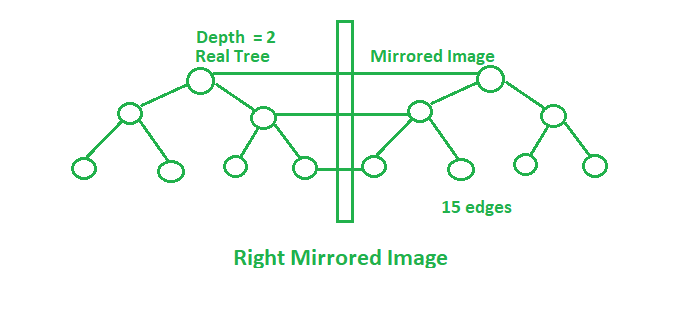# Number of edges in mirror image of Complete binary tree

Given a complete binary tree of depth H. If the mirror image from the left and the right side of this tree is taken then:

Right Mirrored Image: Rightmost node of the every level is connected to mirrored corresponding node.
Left Mirrored Image: Left most node of the every level is connected to mirrored corresponding node.

The task is to find the number of edges after taking both the mirror images in the final tree.Examples:

Input: H = 1
Output: 10
2 edges in the original tree will get mirrored in the mirror images (left and right) i.e. 6 edges in total.
And the edges connecting the mirror images with the original tree as shown in the image above.

Input: H = 2
Output: 24
(6 * 3) + 3 + 3 = 24

## Recommended: Please try your approach on {IDE} first, before moving on to the solution.

Approach: Maintain the leftmost, rightmost nodes after each mirror image. Number of edges will change after each operation of mirror image. Initially,After right mirrored image:After left mirrored image:In complete modified tree:Below is the implementation of the above approach:

## C++

 // C++ implementation of the approach  #include  using namespace std;     // Function to return the total number  // of edges in the modified tree  int countEdges(int H)  {         int edges, right, left;      edges = 2 * (pow(2, H) - 1);      left = right = H + 1;         // Total edges in the modified tree      int cnt = (edges * 3) + left + right;      return cnt;  }     // Driver code  int main()  {      int H = 1;         cout << countEdges(H);         return 0;  }

## Java

 // Java implementation of the approach  import java.io.*;     class GFG {         // Function to return the total number      // of edges in the modified tree      static int countEdges(int H)      {             int edges, right, left;          edges = 2 * (int)(Math.pow(2, H) - 1);          left = right = H + 1;             // Total edges in the modified tree          int cnt = (edges * 3) + left + right;          return cnt;      }         // Driver code      public static void main(String[] args)      {          int H = 1;          System.out.println(countEdges(H));      }  }     // This code has been contributed by anuj_67..

## Python 3

 # Python 3 implementation of the approach     # Function to return the total number  # of edges in the modified tree  def countEdges( H):         edges = 2 * (pow(2, H) - 1)      left = right = H + 1        # Total edges in the modified tree      cnt = (edges * 3) + left + right      return cnt     # Driver code  if __name__ == "__main__":      H = 1;         print(countEdges(H))     # This code is contributed by ChitraNayal

## C#

 // C# implementation of the approach   using System;     class GFG   {          // Function to return the total number       // of edges in the modified tree       static int countEdges(int H)       {              int edges, right, left;                      edges = 2 * (int)(Math.Pow(2, H) - 1);           left = right = H + 1;              // Total edges in the modified tree           int cnt = (edges * 3) + left + right;           return cnt;       }          // Driver code       public static void Main()       {           int H = 1;           Console.WriteLine(countEdges(H));       }      }      // This code is contributed by AnkitRai01

Output:

10


Don’t stop now and take your learning to the next level. Learn all the important concepts of Data Structures and Algorithms with the help of the most trusted course: DSA Self Paced. Become industry ready at a student-friendly price.

My Personal Notes arrow_drop_upCheck out this Author's contributed articles.

If you like GeeksforGeeks and would like to contribute, you can also write an article using contribute.geeksforgeeks.org or mail your article to contribute@geeksforgeeks.org. See your article appearing on the GeeksforGeeks main page and help other Geeks.

Please Improve this article if you find anything incorrect by clicking on the "Improve Article" button below.

Improved By : vt_m, AnkitRai01, chitranayal